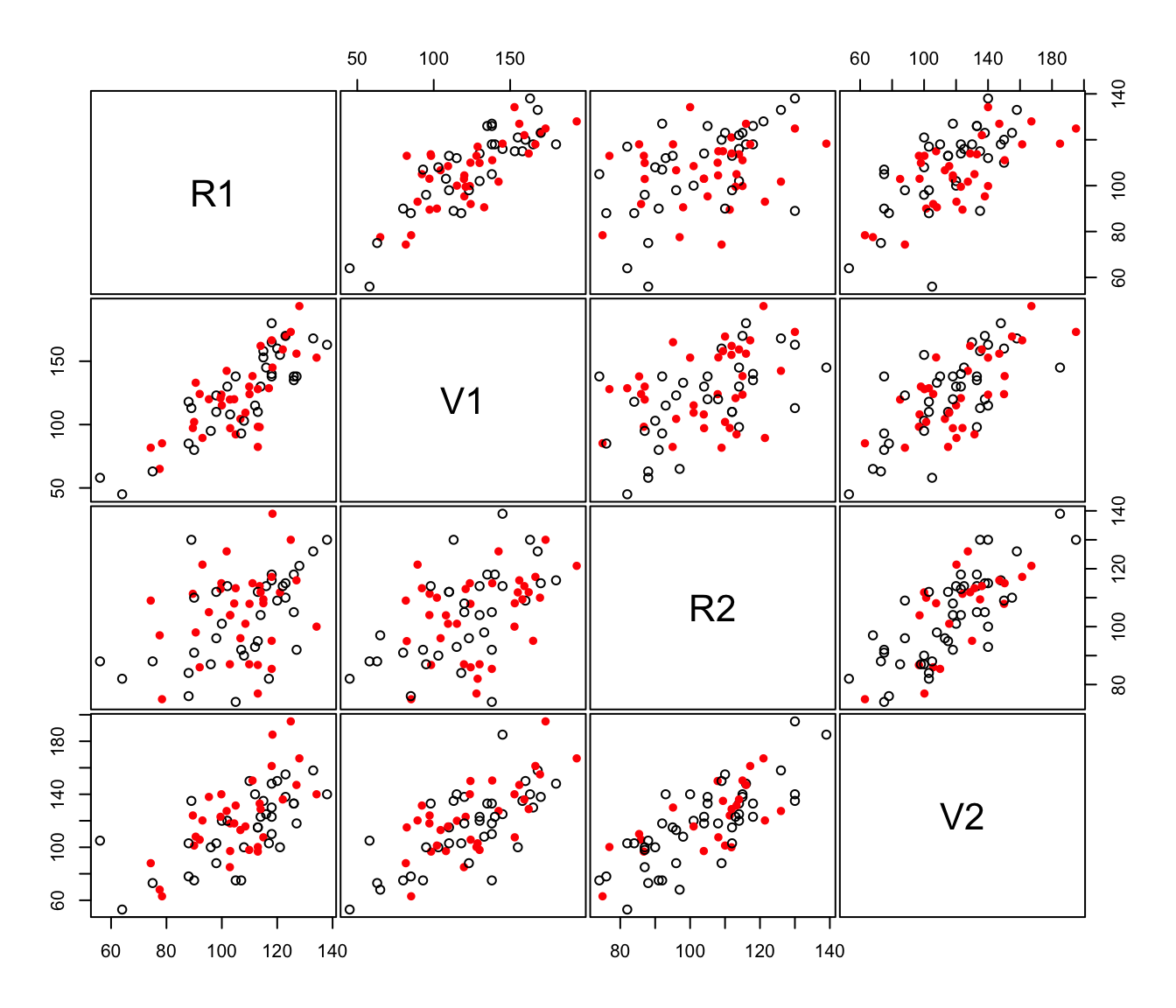Imputes missing data using the mix package.

imputeData(data, categorical = NULL, seed = NULL, verbose = interactive())

Arguments

data

A numeric vector, matrix, or data frame of observations containing missing values. Categorical variables are allowed. If a matrix or data frame, rows correspond to observations and columns correspond to variables.

categorical

A logical vectors whose ith entry is TRUE if the ith variable or column of data is to be interpreted as categorical and FALSE otherwise. The default is to assume that a variable is to be interpreted as categorical only if it is a factor.

seed

A seed for the function rngseed that is used to initialize the random number generator in mix. By default, a seed is chosen uniformly in the interval (.Machine$integer.max/1024, .Machine$integer.max).

verbose

A logical, if TRUE reports info about iterations of the algorithm.

Value

A dataset of the same dimensions as data with missing values filled in.

Examples

# \donttest{
# Note that package 'mix' must be installed
data(stlouis, package = "mix")

# impute the continuos variables in the stlouis data
stlimp <- imputeData(stlouis[,-(1:3)])

# plot imputed values
imputePairs(stlouis[,-(1:3)], stlimp)# }